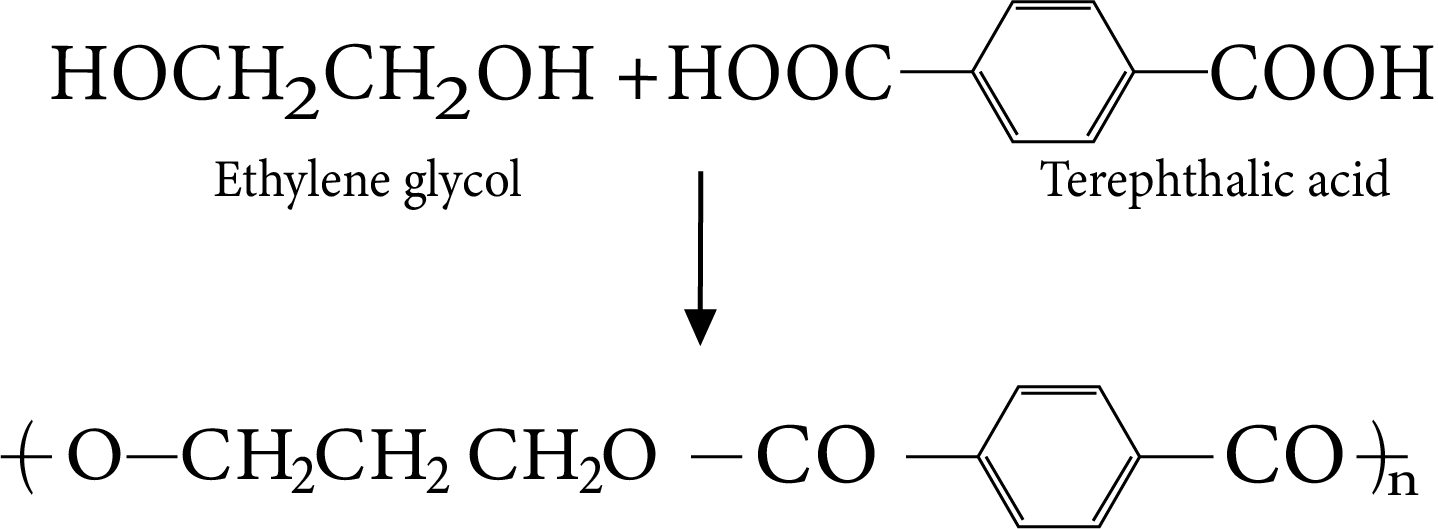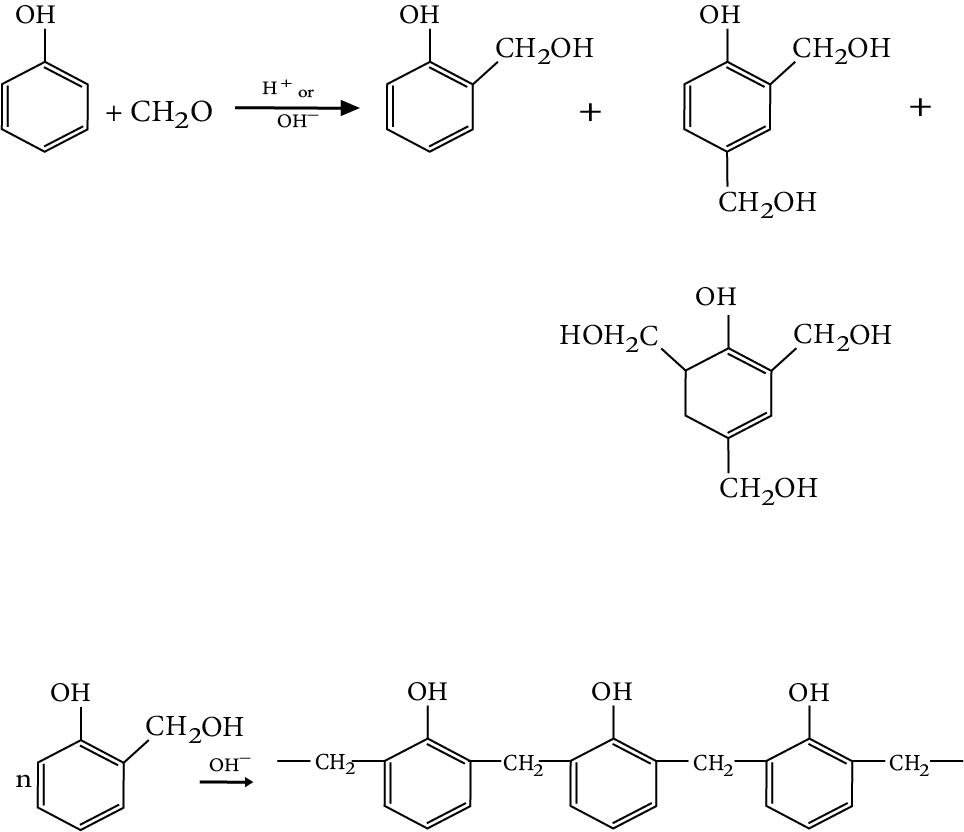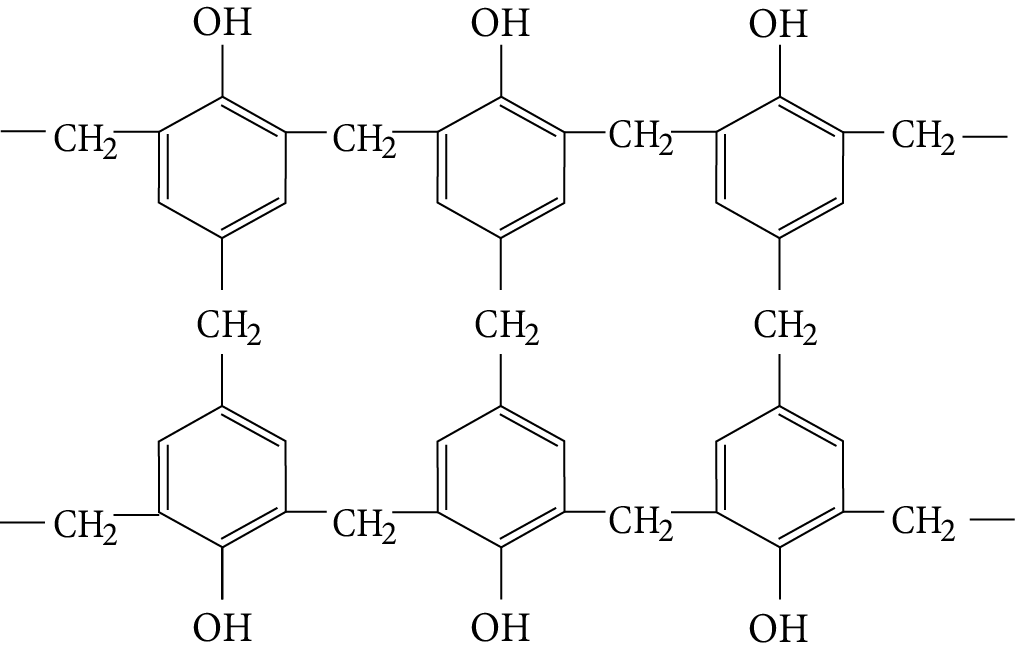Courses
Courses for Kids
Free study material
Offline Centres
More

# Revision Notes on Polymers for NEET 2023Last updated date: 25th Nov 2023
Total views: 159k
Views today: 1.59k

## Revision Notes on Polymers for NEET 2023 - Free PDF Download

Chemistry is one such subject that requires in-depth understanding about chemicals and other aspects of chapters. If you are studying Polymers Class 12 topics, then using notes from Vedantu is the best way to proceed. All equations and chemical formulae are well-explained in Polymers notes for NEET. Students while studying or revising this chapter will get an in-depth understanding about Polymers, Monomers, and other things from these notes. Furthermore, they will also get an easy explanation of Polymers classification. Each portion of the chapter is explained in simple language. If students are finding it challenging to prepare for NEET, they can use Polymers Class 12 NEET notes available in PDF format for free.

These revision Polymers notes are compiled to help students to perform better in NEET. They will learn about chemical formulae, reactions, differences, and other things.

## Access NEET 2023 Revision Notes Chemistry Polymers

### Introduction:

Polymer is defined as a veritably large patch having high molecular mass $({10^{ - 3}} - {10^7}{\text{u}})$.

• Polymers are macromolecules, which are formed by joining or repeating structural units on a large scale.

• This process of conformation of polymer from separate monomers is called polymerisation.

• The metamorphosis of ethene to polythene and commerce of hexamethylene diamine and adipic acid leading to the conformation of Nylon 6, 6 are illustration of two different types of polymerization response

### Classification of Polymers

 S.No Basis of Classification Nature of Polymers Common Example 1 Source of polymer Natural polymersSemisynthetic polymersSynthetic polymers Cotton, silkCellulose rayonPolythene 2 Structure of polymers Linear polymerBranched chainCross linked or network polymer High density polytheneLow density polytheneBakelite melamine 3 Mode of polymerisation Condensation polymerAddition Polymers TerylenePolypropylene, polythene. 4 Molecular force ElastomersFibresThermos plastic PolymersThermosetting polymers cannot be remoulded Buna-NPolyamides such as Nylon-6,6Polythene, PolystyreneBakelite, Urea-HCHO resins 5 Polarity Cationic polymerization polymersAnionic Polymerization Polymers Polystyrene; Polyvinyl ethersBuna type synthetic rubbers 6 Heat treatment Thermoplastic polymersThermosetting Polymer Polyethylene, PVC NyonBakelite, Polysiloxanes 7 Organic and inorganic substance Organic PolymersInorganic Polymers Leather; CelluloseGlass, Silicone rubber

### Classification Based on Growth Polymerisation

The addition and condensation polymers are nowadays also referred to as chain growth polymers and step growth polymers depending on the type of polymerization mechanism they undergo during their formation.

### Types of Polymerisation Reaction

Addition polymerisation or chain growth polymerisation:

• In this type of polymerisation, the molecules of the same monomer or different monomers add together on a large scale form a polymer.

• The monomers used are unsaturated compounds, e.g., alkenes, alkadienyls and their derivation.

• This mode of polymerisation leading to an increase in chain length or chain growth can take place through the formation of either free radicals or ionic species.

When the initiator is cationic in nature, in addition to the double bond, it would generate a cationic intermediate for propagation of the chain process and is termed as cationic addition polymerization.

• The process is initiated by a Lewis acid.

• The important chain initiators used for the cationic polymerisation are $B{F_3},AlC{l_3}$.

• Cation polymerization is facilitated in monomers containing electron-releasing group.

• Isobutylene undergoes cationic polymerization easily as it has two electrons releasing the ${\text{ - C}}{{\text{H}}_3}$ group that will stabilize the intermediate cation.

The reaction proceeds through 3 steps:

• Chain Initiation Step:

${H_2}O + AlC{l_3} \to {H^ + } + [AlC{l_3}{(OH)^ - }]$

• Chain Propagation:

$C{H_3} - {C^ + }HR + C{H_2} = CHR \to C{H_3} - CHR - C{H_2} + {C^ + }HR$

• Chain Termination Step:

${\text{C}}{{\text{H}}_{\text{3}}}{\text{ - CHR - C}}{{\text{H}}_{\text{2}}}{\text{ - }}{{\text{C}}^{\text{ + }}}{\text{HR + n(C}}{{\text{H}}_{\text{2}}}{\text{ = CHR)}}\xrightarrow{{{\text{(AlC}}{{\text{l}}_{\text{3}}}{{{\text{(OH)}}}^{\text{ - }}}{\text{)}}}}{\text{C}}{{\text{H}}_{\text{3}}}{\text{ - CHR - \{ C}}{{\text{H}}_{\text{2}}}{\text{ - CHR}}{{\text{\} }}_{\text{n}}}{\text{ - CH = CHR + }}{{\text{H}}^{\text{ + }}}$

An anion initiator will similar generate carbanionic intermediate and the resulting polymerization is categorized as anionic addition polymerization. Here, the active centre of the propagating species is negatively charged.

• It occurs fluently with monomers containing electron withdrawing groups similar as phenyl, nitrile etc., which are suitable to stabilized the propagation species.

• Inauguration can be brought about by reagents similar as n-butyl lithium or potassium amide.

### The Steps With the Vinyl Derivative Can be Written as Follows:

• Chain Initiation

$Na + N{H_3} \to N{a^ + }NH_2^-$

$NH_2^ - + M \to N{H_2}{M^ - }$

• Chain Propagation

$N{H_2}{M^ - } + (n - 1)M \to N{H_2}M_n^-$

$N{H_2}M_n^ - + N{H_3} \to N{H_2}{M_n}H + NH_2^-$

Here the chain initiator (catalyst) may be benzol peroxide, acetyl peroxide is tert-butyl peroxide, acetyl peroxide is tert-butyl peroxide.

The main steps are involved:

• Chain initiating step

• Chain propagation step

• Chain terminating step

### Condensation Polymerization and Step Growth Polymerisation:

When both the reactants are bifunctional, they undergo a series of condensation reaction in a controlled stepwise manner with the elimination of small molecule like ${H_2}O$, alcohol to form polymers, and the process is called condensation polymerization.

• This process is also called step-growth polymerization’.

• Condensation polymerisation takes place between molecules having polyfunctional groups.

Polyamides: Polymers enjoying amide liaison are important synthetic fibres.

They are made by condensation of diamines with dicarboxylic acid and also from amino acids or caprolactams the cyclic amides.

Polyesters: Dacron is the best known of the polyesters and is manufactured from ethylene glycol and terephthalic acid.

• The reaction is carried out at 420 to 460K in the presence of catalyst mixture of zinc acetate and antimony trioxide.Terylene

• The Terylene fibre (Dacron) is grease resistant and has low moisture absorption.

• It has high tensile strength.

• It is mainly used in making wash and wear garments, in blending with wool to provide better grease and winkle resistance.

### Phenol-Formaldehyde Polymer (Bakelite and Related Polymer)

• Phenol is condensed with formaldehyde in the presence of either an acid or a base.

• The reaction starts with the initial formation of O and/or P-hydroxymethyl phenol derivatives, which further react with phenol to form compounds where rings are joined to each other with -$C{H_2}$ groups.

• The initial product could be a linear product Novolin used in paints.Novolac

On further heating with formaldehyde, novolac undergoes cross-linking to crystallize an infusible common sense called Bakelite.Bakelite

• This polymer called Bakelite is hard, scratch and water resistant.

• It possesses excellent electrical insulating character and hence, it finds major use in making electrical goods.

Copolymerization: If a mixture of more than one monomeric species is allowed to polymerise, a co-polymer is formed and it contains multiple units of each monomer used in the same polymeric chain. The co-polymer can be made not only by chain growth polymerisation, but by step growth polymerisation also.

Co-polymers are further classified into four categories depending on the nature of the different monomers in the chain.

Random Copolymers are formed by the random arrangement of monomer units in the polymer chain.

Alternating Copolymer chains contain the monomer units in alternate.

### Natural Rubber

• It is a natural polymer and possesses remarkable elasticity.

• Latex is a conflation of poly hydrocarbon driblets in a waterless result (oil in water emulsion). It consists of negatively charged particles of rubber. The latex contains about 35% rubber. The rubber present in the latex is coagulated by the addition of ${\text{C}}{{\text{H}}_{\text{3}}}{\text{COOH}}$ or ${\text{HCOOH}}$

• This solid is crude rubber: This is refined by

1. Break down i.e., masticating the raw rubber and

2. Compounding i.e., the addition of necessary agents to the rubber

### Structure of Rubber:

• The empirical formula of Natural rubber is ${{\text{C}}_{\text{5}}}{{\text{H}}_{\text{8}}}$.

• Natural rubber may be considered as a linear 1,4- addition polymer of isoprene.

• The residual double bonds are located between ${{\text{C}}_{\text{2}}}$ and ${{\text{C}}_{\text{3}}}$ of isoprene units in the polymer. the position of double bonds is detected by ozonolysis.

All these double bonds have cis configuration and thus, rubber is cis 1,4 - polyisoprene.

### Vulcanization of Rubber

Natural rubber becomes soft at high temperatures (>335K) and brittle at low temperatures (< 283 K) and shows high water absorption capacity. It is soluble in nonpolar solvents and is non-resistant to attack by oxidising agents.

• Addition of sulphur to hot rubber causes changes that improve its physical properties. This process is called Vulcanization.

• It was performed by heating a mixture of raw rubber and sulphur at 373 to 415 K.

• This process is slow and additives such as zinc oxide, zinc stearate etc., are used to accelerate the rate of vulcanization.

• The Vulcanized rubber has excellent elasticity, low water- absorption tendency, resistant to oxidation and organic solvents.

### Synthetic Rubber:

• The Polymers derived from butadiene derivatives and contain carbon-carbon double bonds so that they can also be vulcanized.

• Synthetic rubbers are either homopolymers of buta -l, 3 - diene derivatives or copolymers, of which one of the monomers is buta -1, 3 - diene. Commonly used synthetic rubbers are Buna-S, Buna-N, Neoprene and Butyl rubber.

### Difference Between Natural and Synthetic Polymers:

 S.No Distinguishing property Natural Polymers Synthetic Polymers 1 Source of their preparation and common example Obtained in natural wool, silk, jute, etc Prepared in the lab, nylon, terrene, Dacron etc. 2 Length of polymer chain Not of uniform length Can be of uniform length 3 Affinity for sulphur and vat dyes Posses very high affinity Possess low affinity 4 Fixing quality Low High

### Determination of Molecular Mass of Polymers

The Molecular mass of a polymers can be expressed in two ways:

1. Number average molecular mass $({\bar M_n})$

If ${N_1},{N_2},{N_3},......$are the number of molecules with molecular masses ${M_1},{M_2},{M_3},......$respectively, the number average molecular mass is ${\bar M_n} = \dfrac{{{N_1}{M_1} + {N_2}{M_2} + ....... + {N_i}{M_i}}}{{{N_1} + {N_2} + {N_3} + ........ + {N_I}}} = \dfrac{{\sum\limits_{i = 1}^n {{N_i}{M_i}} }}{{\sum\limits_{i = 1}^n {{N_i}} }}$.

${\bar M_n}$ is determined by

• End group analysis method

• Colligative properties like osmotic pressure

${\bar M_n}$ is related to osmatic pressure by the equation

• ${\left( {\dfrac{\pi }{C}} \right)_0} = RT.\dfrac{1}{{\bar Mn}}$ or $\bar Mn = {\left( {\dfrac{C}{\pi }} \right)_0}RT$

C is concentration of polymer solution in gm/litre is osmatic pressure in cms

• ${\left( {\dfrac{\pi }{c}} \right)_0}$value is calculated from the graph plotted between $\dfrac{\pi }{c}$on y axis and C on X-axis by extrapolated technique, the graph is straight line with an intercept is $\dfrac{{RT}}{{\bar Mn}}$on y axis

1. Weight average molecular mass $({\bar M_w})$.

When the total of groups of molecules, having different molecular are multiplied with their all the, molecules, the result obtained is called the   or weight average molecular

${\bar M_w} = \dfrac{{{N_1}M_1^2 + {N_2}M_2^2 + ....... + {N_i}M_i^i}}{{{N_1}{M_1} + {N_2}{M_1} + ........ + {N_i}{M_i}}} = \dfrac{{\sum\limits_{i = 1}^n {{N_i}M_i^2} }}{{\sum\limits_{i = 1}^n {{N_i}{M_i}} }}$

${N_1},{N_2},{N_3},......$ are  number of molecules

${M_1},{M_2},{M_3},......$are molecular masses

Weight average molecular weight is determined by

• Light scattering

• Ultra-Centrifugal method

• Viscosity measurements

PDI: The ratio of weight average molecular mass to the number average molecular rms is called Poly dispersity index(PDI).

$PDI = \dfrac{{{{\bar M}_w}}}{{{{\bar M}_n}}}$

• The value of PDI for polymers in between 1 and 1.5.

• For natural polymers, PDI is usually unit (natural polymers and mono dispersed) $({\bar M_w} = {\bar M_n})$.

• For synthetic polymer, PDI is greater than one $({\bar M_w} > {\bar M_n})$.

• ${\bar M_n}$ is measured on the basis of the colligative properties.

• The mass average molecular mass ${\bar M_w}$ is measured with the help of ultra-centrifugations, sedimentation etc.

• These are polymers which are degraded by microorganisms with in a suitable period so that the polymers and their degraded products do not cause any serious effects on the environment. Biopolymers degrade mainly by enzymatic hydrolysis and oxidation.

• These synthetic polymers mostly have functional groups which are normally present in biopolymers and lipids.

## Importance of Polymers Class 12 Chapter

In the chapter, students will learn about Polymers which are materials with a high molecular mass and a large number of repeating structural units. Polymers are also known as macromolecules since they are single, massive molecules, i.e. large molecules. The notes further describe classification of Polymers which includes- Natural Polymer, Synthetic Polymers, and Semi Synthetic Polymers. The further classification is based on the structure which includes Linear Polymers, Branched Chain Polymers, and Cross Linked or Network Polymers.

Students while learning the chapter will gain knowledge based on the molecular forces and in different steps. There is an important addition in Polymers which involves the condensation reaction between two bi-functional monomers is repeated. In condensation processes, the product of each step is a bi-functional species, and the condensation sequence continues. This technique is also known as step growth polymerization since each stage produces a distinct functionalised species that is independent of the others.

Steps, formulae and classifications taught in this chapter are quite challenging to understand, especially during the last minute revision period. Thanks to polymers Class 12 NEET notes with easy presentation of each area, you can ace the chapter in no time. The chapter creates a strong foundation for polymers in different angles.

## Benefits of Vedantu's Polymers Class 12 Notes

• These NEET revision notes are created by Vedantu's top chemistry subject experts to simplify the explanation of all the formulae in this chapter. The fundamental concept of defining chemical reactions will become much more obvious to you.

• Use these notes to quickly finish preparing this chapter. Once you have these notes from Vedantu, you don't need any other resources for last-minute NEET revision.

• Add these notes to your study sessions to quickly learn the classification and types. You can study this chapter more effectively if you use these notes.

Learn from the experts by downloading these notes in a free PDF format. Use these notes to quickly recall everything you've learned in this chapter. Using the Polymer Chemistry notes PDF, you can learn how experts have solved queries. Chemistry can be challenging since the same issue might provide multiple answers. Using the PDF file, determine the best technique and get the correct answers.

### Other Important Links Related to NEET Polymers

 Other Important Links for NEET Polymers NEET Polymers Important Chapter NEET Polymers Important Questions

## FAQs on Revision Notes on Polymers for NEET 2023

1. What are Polymers in Chemistry Class 12?

Polymers are macromolecules with a high molecular mass that are made up of repeating structural units formed from the right monomers. A monomer is the unit molecule that combines with another resulting in the formation of another polymer. Polymerization refers to the process of forming polymers from monomers.

2. What are different sections of polymers?

Elastomers: The weakest intermolecular forces hold polymer chains together. Polymers are solids having rubber-like characteristics.

Thermoplastic Polymers: These Polymers are kept together by intermolecular forces that are similar to those of elastomers and fibres. These polymers can soften and harden repeatedly when heated and cooled.

Addition Polymerization: Addition polymerization is also known as chain growth polymerization because it occurs in steps, each of which provides reactive intermediates for use in the next stage of chain growth.

3. What are the properties of polymers?

Some of the properties of Polymers are- Crystallinity, Permeability, Elastic Modulus, and others.

4. Why are polymers important in industry?

Industrial polymers are chemical substances that are employed in the production of synthetic industrial products. A wide range of polymers are utilised in the commercial production of plastics, elastomers, adhesives, and surface coatings.